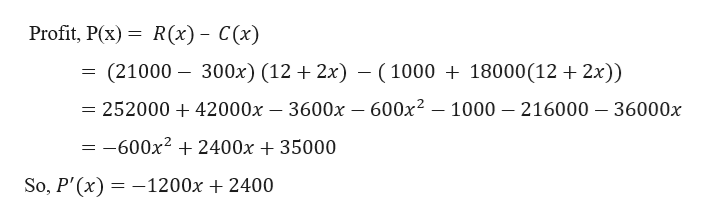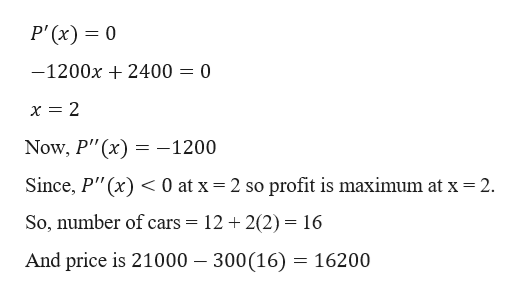# An automobile dealer can sell 12 cars per day at a price of \$21,000. He estimates that for each \$300 price reduction he can sell two more cars per day. If each car costs him \$18,000, and fixed costs are \$1000, what price should he charge to maximize his profit? [Hint: Let x = the number of \$300 price reductions.]How many cars will he sell at this price?

Question
81 views

An automobile dealer can sell 12 cars per day at a price of \$21,000. He estimates that for each \$300 price reduction he can sell two more cars per day. If each car costs him \$18,000, and fixed costs are \$1000, what price should he charge to maximize his profit? [Hint: Let x = the number of \$300 price reductions.]

How many cars will he sell at this price?

check_circle

Step 1

Given,

Number of cars sell in a day = 12

Price of a car is \$21,000.

For each car \$300 price reduction if he can sell two more cars per day.

Let be x unit 300 price reduction.

2x units increases in sale of car

Price = 21000 – 300x

Car sold = 12 + 2x

R(x) = (21000- 300x) (12+2x)

Cost; C(x) = 1000 + 18000(12+2x)

Thenhelp_outlineImage TranscriptioncloseProfit, P(x) R(x) C(x) (21000 300x) (12 2x) -100018000(12 2x)) = 252000 + 42000x - 3600x - 600x2 - 1000 216000 -36000x = -600x2 2400x 35000 So, P'(x) 1200x 2400 fullscreen
Step 2

For critical ...help_outlineImage TranscriptioncloseP' (x) 0 -1200x2400 = 0 Now, P"(x)-1200 profit is maximum at x 2 Since, P"(x) 0 at x 2 so So, number of cars = 12 2(2) = 16 And price is 21000 - 300(16) = 16200 fullscreen

### Want to see the full answer?

See Solution

#### Want to see this answer and more?

Solutions are written by subject experts who are available 24/7. Questions are typically answered within 1 hour.*

See Solution
*Response times may vary by subject and question.
Tagged in

### Calculus